# Solving a second-order differential equation

Dear all,

There is another error in our differential equation.

Starting over from part e) we solve the following equation for (dv/dt)2.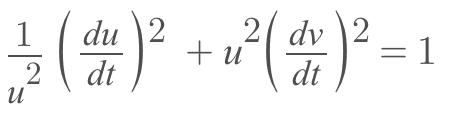giving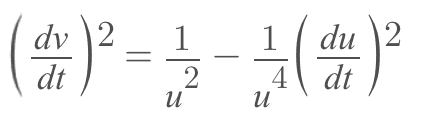and substitute it in the following equation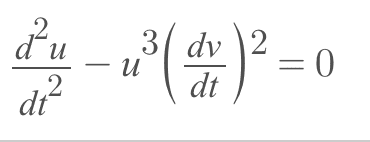giving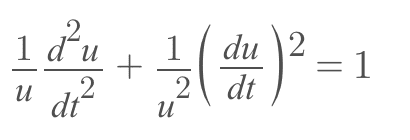Which offers no clear way to solve. We examine the following expression from part d).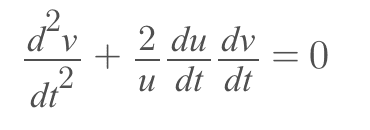which makes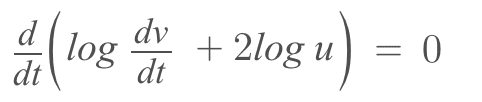which implies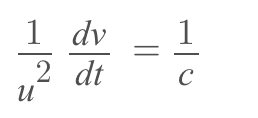for some constant c. solving,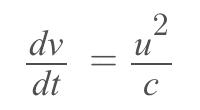substitute into the first equation to get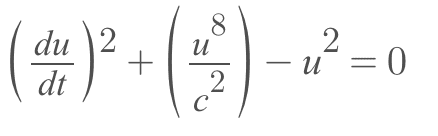which makes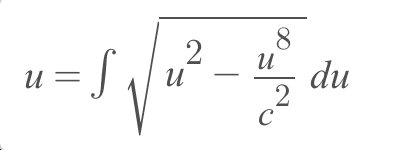I think this way is not easy.

Last edited:
The expression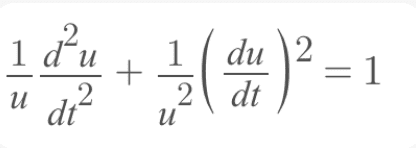has the solution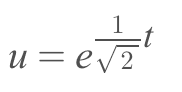and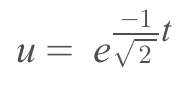For the v component of the geodesic, we plug in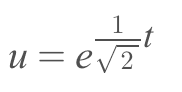into the unit speed equation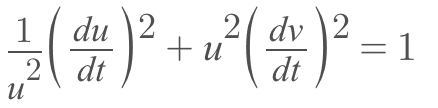giving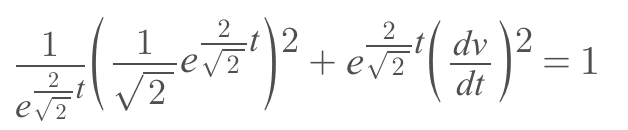and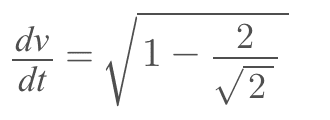We then integrate to give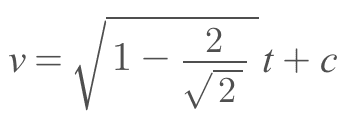and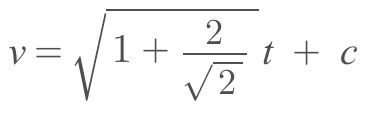which is incorrect judging from the following plot in the uv plane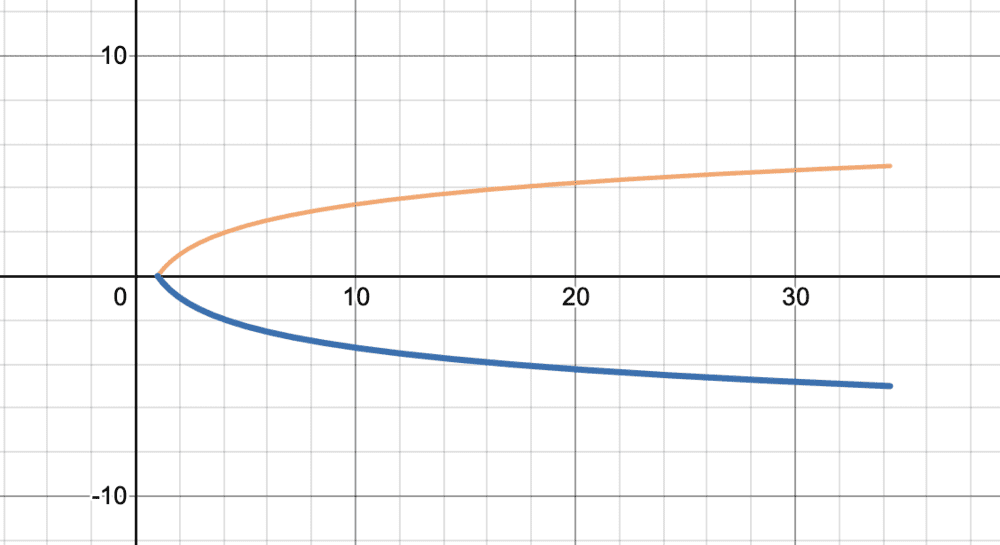but I think we are getting closer to the correct solution.

Last edited:
haruspex
Homework Helper
Gold Member
2020 Award
•docnet
What course is this? Differential geometry?

You seem to have squared part of ##\frac{du}{dt}## twice. The left hand term above should reduce to 1/2.

I am sorry I should apologize because the solution to our ODE is not a geodesic, because we are solving the wrong ODE.

Not solving this problem has been eating me up. So, I revisited our previous calculations tonight, made sure to be extra careful not to make errors and ended up with the following. I still have not learned how to write LaTex equations, so these were typed in Microsoft Word, and these will not show up in quoted messages.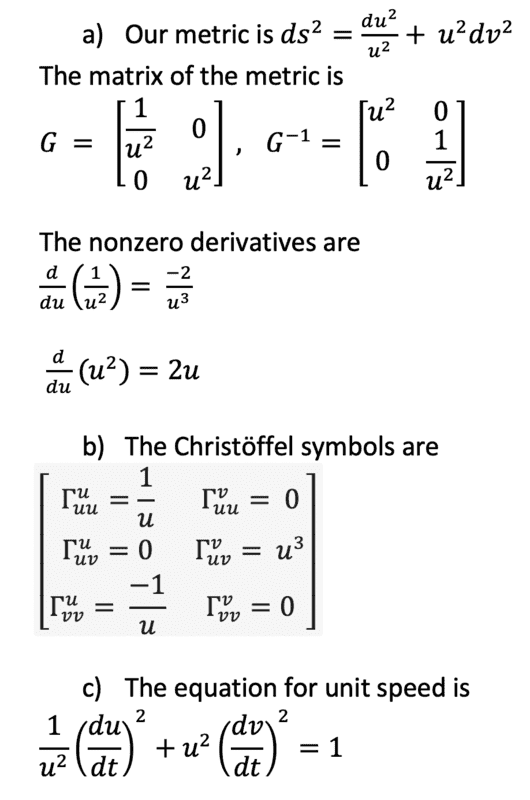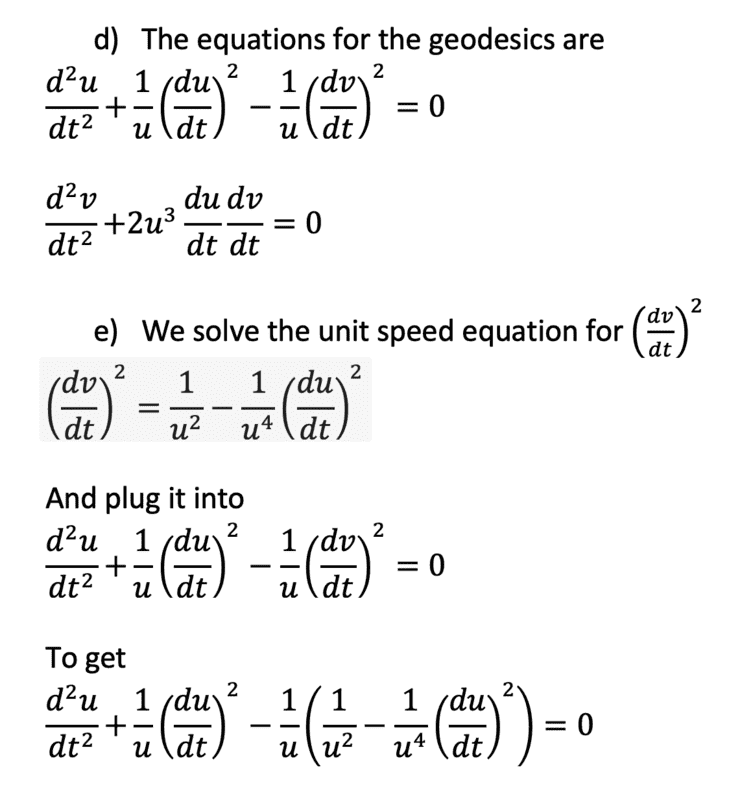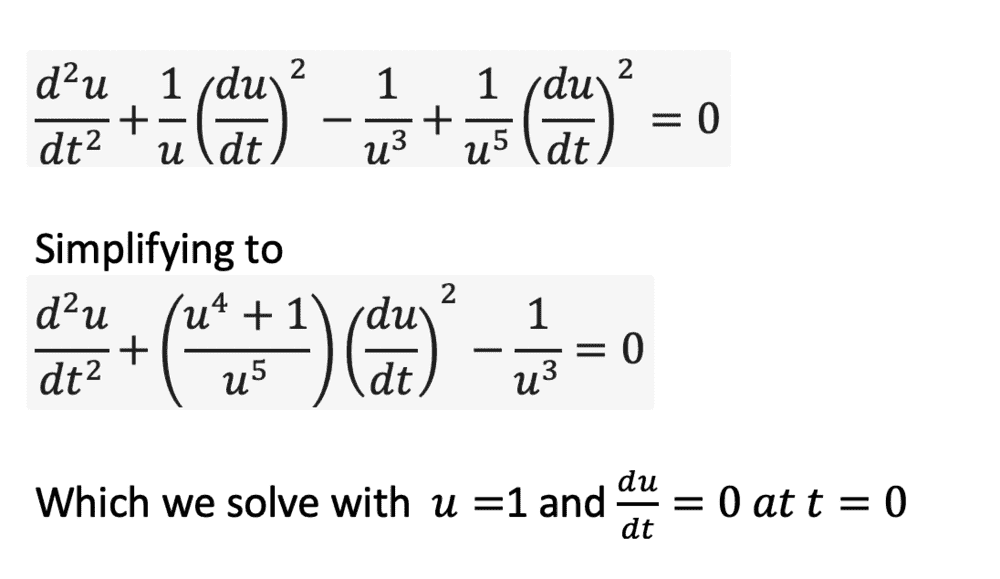I am unable to solve this nonlinear second order ODE by any method I am familiar with.

Is anyone able to compute it using Mathematica or Wolfram Αlpha?

Thank you.

Last edited:
Acccording to DSolve in Mathematica, the fearful ODE $$\frac{d^2u}{dt^2}=\frac{1}{u^3}-\Big(\frac{u^4+1}{u^5}\Big)\Big(\frac{du}{dt}\Big)^2$$
has "closed form" solutions given by...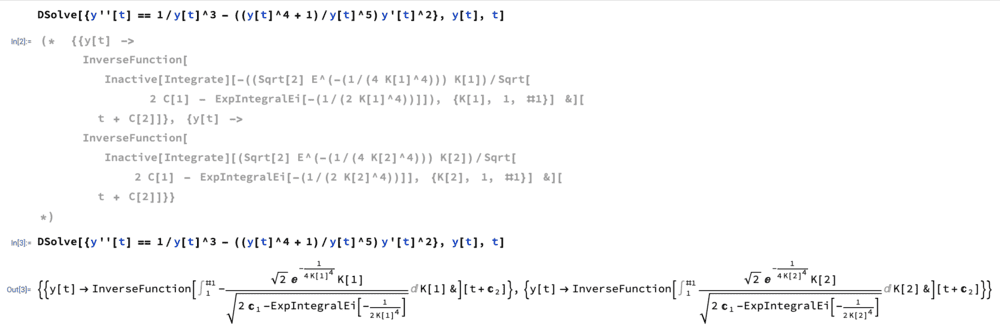By using DSolve with initial value conditions,

$$\frac{d^2u}{dt^2}=\frac{1}{u^3}-\Big(\frac{u^4+1}{u^5}\Big)\Big(\frac{du}{dt}\Big)^2\Rightarrow u(t)=\sqrt{1+t^2}$$

(g) we plug ##u(t)## into the unit speed equation to find
$$\frac{dv}{dt}=\Big(\frac{t^4+t^2+1}{(1+t^2)^3}\Big)^{\frac{1}{2}}$$

which is a separable equation, when trying to compute
$$v(t)=\int \Big(\frac{t^4+t^2+1}{(1+t^2)^3}\Big)^{\frac{1}{2}}dt$$

we cannot find an explicit closed form solution